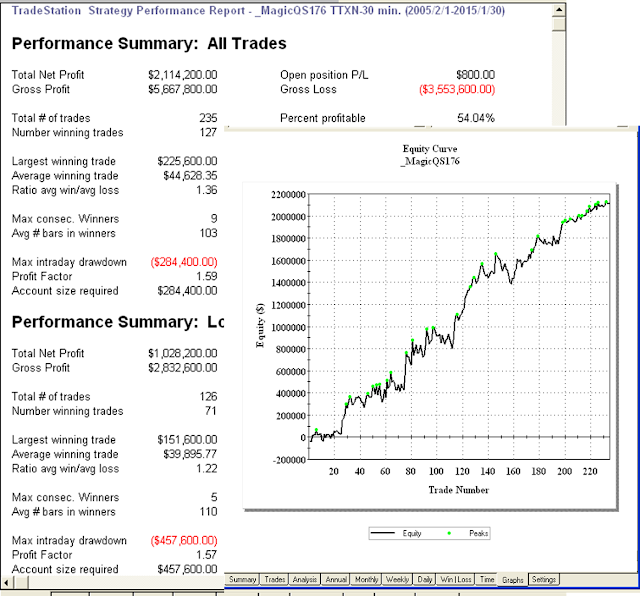## 2015年6月4日 星期四

### 開發商品的交易系統 - 基礎篇 

Inputs: Price(Close), Length1(2),Length2(5),Length3(7);
Plot1(RateofChange(Price, Length1) + (RateofChange(Price, Length2) + (RateofChange(Price, Length3))/3));

Step 1： 確認此標的是否為較強勢的標的。原作者簡單採用上面的選市場方式。
Step3： 用一個簡單的指標判定多空，作者使用MACD指標，在0軸以上才作多。
Step6：最近是否突破近期高點。 →型態上的確認。

1、ROC的參數
ROC中只含一個參數,那就是究竟是同多少天之前的股價進行比較,這個天數N就是參數。

ROC的應用法則

1) 從ROC的取值方面
ROC自上而下跌破0,是賣出信號。反之，ROC自下而上穿過0,是買進信號。這是由ROC描述股價變動速度的特性而定的。

2) 從ROC與ROCAVG的相對取值方面

3) 從ROC與股價的背離方面
ROC有領先於股價的特性,所以有如下的法則。{系統參數與變數}
input:EntryType(1),ExitType(1);
vars: IsBalanceDay(False),MP(0),PF(0),PL(0);

inputs: Length1(3),R2(2.3),R3(2.2),HighBar(3),LowBar(3);
Vars:Price(0),Length2(5),Length3(7),MyROC(0);

MP = MarketPosition ;
if DAYofMonth(Date) > 14 and DAYofMonth(Date) < 22 and DAYofWeek(Date)= 3 then isBalanceDay = True else isBalanceDay =False ;

{ 利用短中長週期平均值來建立較均化的變動率}
Price = Close ;
Length2 = IntPortion(Length1 * R2) ;
Length3 = IntPortion(Length2 * R3) ;

MyROC = (RateofChange(Price, Length1) + RateofChange(Price, Length2) + RateofChange(Price, Length3))/3;

{ MACD 作為趨勢方向確認 }
Value2 = Macd(Close,12,26) ;

{ 當變動率突破其長週期均值，且 MACD在零軸上，以最近高點突破進場}
if MP <> 1 and MyROC Cross over Average(MyROC,Length3) and Value2 > 0 then buy next bar at Highest(High,Length3) stop ;

{ 當變動率跌破其長週期均值，且 MACD在零軸下，以最近低點跌破進場}
if MP <> -1 and MyROC Cross under Average(MyROC,Length3) and Value2 < 0 then sell next bar at Lowest(Low,Length3) stop;

if ExitType = 1 then SetStopLoss(PL * BigPointValue) ;

if ExitType = 2 then Begin
SetStopLoss(PL * BigPointValue) ;
setProfitTarget(PF * BigPointValue) ;
end;

if ExitType = 3 then Begin
if MP > 0 and BarsSinceEntry = NBarL then ExitLong next bar at Market ;
if MP < 0 and BarsSinceEntry = NBarS then ExitShort next bar at Market ;
end;

if ExitType = 4 then Begin
SetStopLoss(PL * BigPointValue) ;
setProfitTarget(PF * BigPointValue) ;
if MP > 0 and BarsSinceEntry = NBarL then {Sell } ExitLong next bar at Market ;
if MP < 0 and BarsSinceEntry = NBarS then {Buy} ExitShort next bar at Market ;
end;

if IsBalanceDay or date = 1150224 then setExitonClose ;MagicQS176